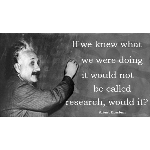Community Profile# Jack Sparrow

16 total contributions since 2013

View details...

Contributions in
View by

Question

adding multiple images together from a for loop
I am trying to adding multiple images together into one in a for loop, but seem to only display the last image. This is what I'v...

6 years ago | 1 answer | 0

### 1

Question

Determine lowest part in sub regions of image and segment the image
I want to segment object into head, torso and leg region. There is a binary image and I want to: - Calculate the sum of wh...

7 years ago | 1 answer | 0

### 1

How to calculate the Euclidean distance between two fingerprint images?
I think euclidean distance formula is: d(H1,H2) = sqrt((H1(i) - H2(i))^2) H1 = probe set H2 = gallery set

7 years ago | 0

Question

Need help on creating loop
Please I need assistance on this code, I am trying to matching two data. The first one is the training set, while the second one...

7 years ago | 0 answers | 0

### 0

Question

Facial shape extraction from images
What suitable algorithms can be applied for extracting shape of the face for face recognition, I thought of using edge detection...

7 years ago | 1 answer | 0

### 1

labeling target in neural network in matlab
Thank you Greg, This is quite complicated than I thought. What if I set the label of the 5 samples of the first subject to 1 and...

7 years ago | 0

Question

labeling target in neural network in matlab
I have a training set of 200rows, 600columns as the training set, each 5 rows represent feature vectors from one subject, meanin...

7 years ago | 3 answers | 0

### 3

Question

Decision fusion of multiple features from small data set
This is not a much specific question, I just want to gather idea from colleagues in this forum. My question is; I estimate...

7 years ago | 0 answers | 0

### 0

Question

How to return the height of a bounding box
How can I return the height of a bounding box of an object to the work space from an object detection algorithm like Gaussian Mi...

7 years ago | 1 answer | 0

### 1

Question

how to plot top and bottom points in object detection
I am using the Gaussian Mixture Model to detect object (human) in a video. but, I want to plot the head and feet position of the...

7 years ago | 0 answers | 0

### 0

Question

How to divide an image in two blocks
How can I divide an image into two halves? Then, after dividing it make each half independent, say A = top half and B = t...

7 years ago | 2 answers | 0

### 2

Question

issue with coefficients of Discrete wavelet transform
Please why am I getting 1 x 10517 as the result of coefficient exported to the workspace after using Discrete Wavelet Transform....

7 years ago | 1 answer | 0

### 1

Question

wavelet transform for feature vector
Can someone please explain what the coefficients from Discrete wavelet transform are for and how can they be used to form featur...

7 years ago | 1 answer | 0

### 1

Question

Height and Weight Estimation
Can someone please help me out on how to do human height and weight estimation in still images. If possibly algorithms related. ...

7 years ago | 1 answer | 0

### 1

Question

IDENTIFY COLOR IN NIR
I'm working on a project, i need to identify the ethnicities from the images but they are all taken in near infrared. so i'm con...

7 years ago | 2 answers | 0